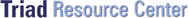#### Data Comparibility

The comparability of different data sets determines how they can be used collectively to support decision-making.

Data comparability is one of the characteristics used to describe analytical data quality. The concept of data comparability is particularly critical to the Triad for two reasons. First, the Triad emphasizes collaborative data sets. Collaborative data sets, by definition, contain data from different analytical methods. The comparability of different data sets determines how they can be used collectively to support decision-making. Second, "comparability" is also often used to convey a measure of data usability for techniques of less rigorous analytical quality. In these cases the point of comparison is usually a standard fixed-laboratory method. Depending on how "comparability" is defined and measured, these types of comparisons can be used incorrectly as the basis for rejecting real-time data that are, in fact, of value for decision-making purposes.

There are a number of factors that contribute to, or detract from, data comparability. These can be grouped into two general categories, factors related to sample collection and handling, and factors related to the analytical methods used. Sample collection issues include sample support (i.e., exactly what was sampled) and acquisition techniques, environmental conditions at the time of sampling, and sample handling/preservation methods. Gamma spectroscopy measurements for radionuclides provide an example of how sample support affects data comparability. Gamma spectroscopy is a standard fixed-laboratory method for identifying and quantifying activity concentrations for many common radionuclides. The same systems, with some adjustments, can also be deployed in the field and used to measure activity concentrations in situ. However, one would never expect the results from a soil sample laboratory measurement to be directly comparable with those of a direct measurement obtained at the soil sample location. This is because the laboratory method measures average activity concentrations in approximately 400g of soil, while the direct measurement would measure a spatially weighted-average activity concentration for several tons of soil. Unless sample support, acquisition, handling, and preservation techniques are identical for two different methods, one should not expect resulting data to be directly comparable.

Analytical issues related to data comparability include sample preparation, cleanup, and determinative methods used. A very basic example of a comparability issue is the degree to which a sample has been homogenized and/or segregated with portions removed (e.g., organic matter, stones, etc.) before sub-samples are obtained. A second example is the degree to which digestion has taken place for those analyses that include an extraction step. In the case of determinative methods, differing detection levels and/or levels of QA/QC can affect comparability even if the same determinative method was used. As with sampling issues, unless analytical issues associated with data comparability are controlled there is no reason to expect data to be quantitatively comparable.

One common means for evaluating data comparability (or the lack of it) is through the use of split samples and regression analysis or correlation coefficients. In the case of regression analysis, the adjusted coefficient of determination is often quoted as a measure of comparability. In the case of correlation coefficients, it is the correlation coefficient itself that measures the linear relationship between two sets of analytical results derived from sample splits.

In the case of comparability and the Triad, it is important to remember the following points:

• The term "comparability" is an umbrella term that encompasses an array of sampling and analysis characteristics that individually may or may not be comparable when contrasting two different sampling/analytical procedures and their results.

• The lack of comparability between two data sets derived from different sampling/analytical procedures does not necessarily mean that one of the data sets is inferior from a decision support perspective. In particular, poor regression results or low correlation coefficients arising from a statistical analysis of sample split analyses do not automatically mean that an alternative sampling/analytical protocol cannot be used for decision-making purposes.

• The level of comparability between two different sampling/analytical procedures does affect the way data sets can be combined, interpreted, and used collaboratively to support decision-making. For example, for data sets with a high degree of comparability it may be possible to quantitatively combine data sets for decision-making purposes. For data sets with a low degree of comparability, data from different sources may need to be treated in a weight-of-evidence manner to support decisions.